# 告别动态规划，连刷 40 道题，我总结了这些套路，看不懂你打我（万字长文）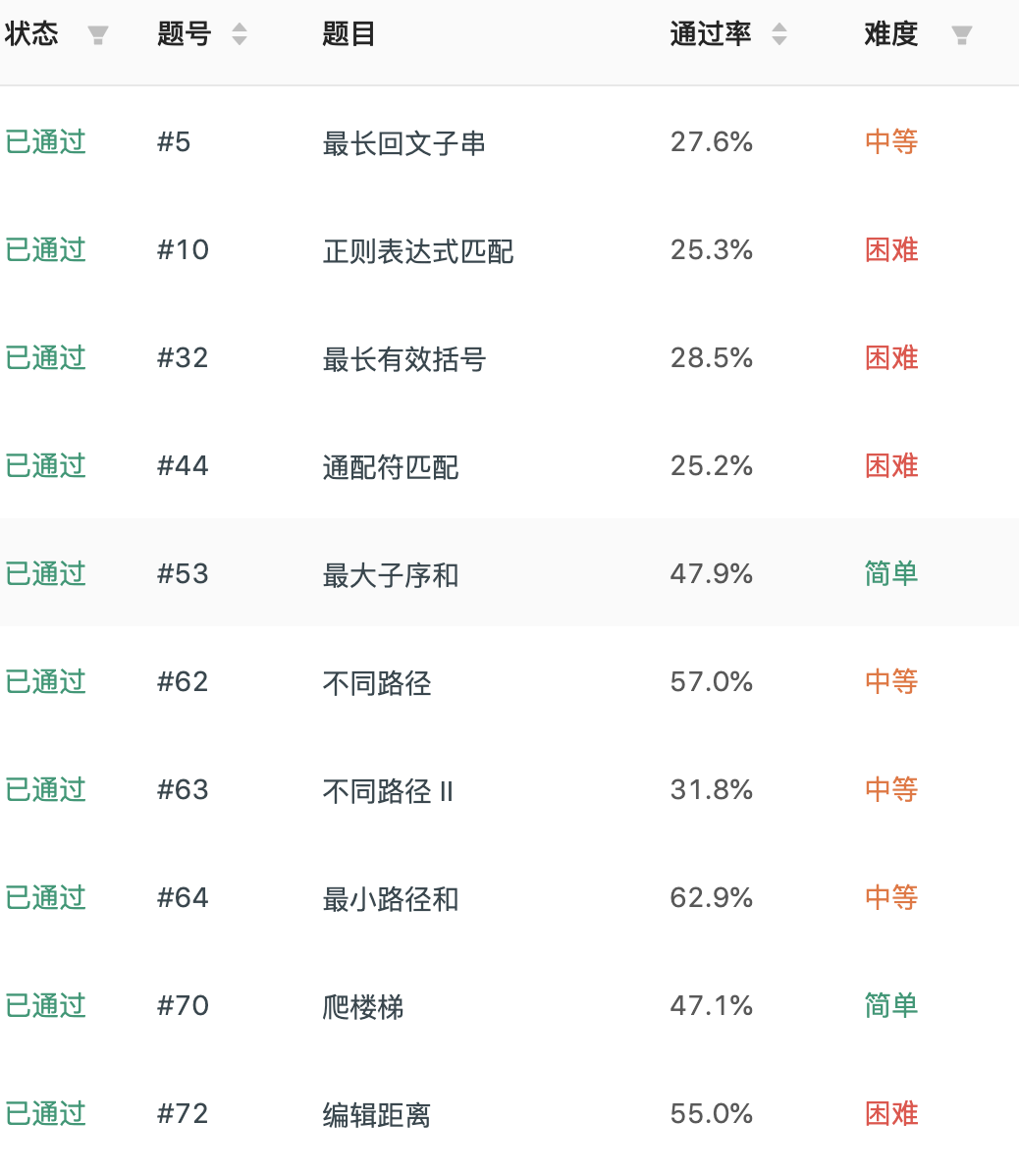### 二、案例详解

#### 案例一、简单的一维 DP

##### （3）、找出初始条件

dp = 0.
dp = 1.

int f( int n ){
if(n <= 1)
return n;
// 先创建一个数组来保存历史数据
int[] dp = new int[n+1];
// 给出初始值
dp = 0;
dp = 1;
// 通过关系式来计算出 dp[n]
for(int i = 2; i <= n; i++){
dp[i] = dp[i-1] + dp[-2];
}
// 把最终结果返回
return dp[n];
}


#### 案例二：二维数组的 DP

##### 问题描述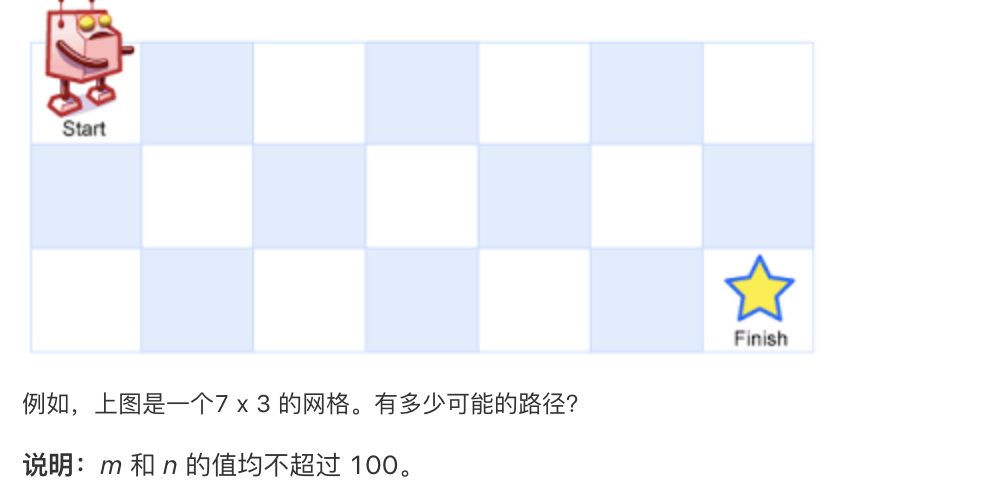##### 步骤三、找出初始值

dp [0….n-1] = 1; // 相当于最上面一行，机器人只能一直往左走

dp[0…m-1]  = 1; // 相当于最左面一列，机器人只能一直往下走

##### 撸代码

public static int uniquePaths(int m, int n) {
if (m <= 0 || n <= 0) {
return 0;
}

int[][] dp = new int[m][n]; //
// 初始化
for(int i = 0; i < m; i++){
dp[i] = 1;
}
for(int i = 0; i < n; i++){
dp[i] = 1;
}
// 推导出 dp[m-1][n-1]
for (int i = 1; i < m; i++) {
for (int j = 1; j < n; j++) {
dp[i][j] = dp[i-1][j] + dp[i][j-1];
}
}
return dp[m-1][n-1];
}


O(n*m) 的空间复杂度可以优化成 O(min(n, m)) 的空间复杂度的，不过这里先不讲

#### 案例三、二维数组 DP

##### 问题描述

举例：

arr = [
[1,3,1],
[1,5,1],
[4,2,1]
]



##### 步骤二：找出关系数组元素间的关系式

dp[i] [j] = min(dp[i-1][j]，dp[i][j-1]) + arr[i][j];// arr[i][j] 表示网格种的值

##### 步骤三、找出初始值

dp [j] = arr [j] + dp [j-1]; // 相当于最上面一行，机器人只能一直往左走

dp[i]  = arr[i]  + dp[i] ; // 相当于最左面一列，机器人只能一直往下走

##### 代码如下
public static int uniquePaths(int[][] arr) {
int m = arr.length;
int n = arr.length;
if (m <= 0 || n <= 0) {
return 0;
}

int[][] dp = new int[m][n]; //
// 初始化
dp = arr;
// 初始化最左边的列
for(int i = 1; i < m; i++){
dp[i] = dp[i-1] + arr[i];
}
// 初始化最上边的行
for(int i = 1; i < n; i++){
dp[i] = dp[i-1] + arr[i];
}
// 推导出 dp[m-1][n-1]
for (int i = 1; i < m; i++) {
for (int j = 1; j < n; j++) {
dp[i][j] = Math.min(dp[i-1][j], dp[i][j-1]) + arr[i][j];
}
}
return dp[m-1][n-1];
}


O(n*m) 的空间复杂度可以优化成 O(min(n, m)) 的空间复杂度的，不过这里先不讲

#### 案例 4：编辑距离

示例：

horse -> rorse (将 'h' 替换为 'r')
rorse -> rose (删除 'r')
rose -> ros (删除 'e')


##### 步骤二：找出关系数组元素间的关系式

（1）、如果把字符 word1[i] 替换成与 word2[j] 相等，则有 dp[i] [j] = dp[i-1] [j-1] + 1;

（2）、如果在字符串 word1末尾插入一个与 word2[j] 相等的字符，则有 dp[i] [j] = dp[i] [j-1] + 1;

（3）、如果把字符 word1[i] 删除，则有 dp[i] [j] = dp[i-1] [j] + 1;

dp[i] [j] = min(dp[i-1] [j-1]，dp[i] [j-1]，dp[[i-1] [j]]) + 1;

##### 代码如下
public int minDistance(String word1, String word2) {
int n1 = word1.length();
int n2 = word2.length();
int[][] dp = new int[n1 + 1][n2 + 1];
// dp[0...n2]的初始值
for (int j = 1; j <= n2; j++)
dp[j] = dp[j - 1] + 1;
// dp[0...n1] 的初始值
for (int i = 1; i <= n1; i++) dp[i] = dp[i - 1] + 1;
// 通过公式推出 dp[n1][n2]
for (int i = 1; i <= n1; i++) {
for (int j = 1; j <= n2; j++) {
// 如果 word1[i] 与 word2[j] 相等。第 i 个字符对应下标是 i-1
if (word1.charAt(i - 1) == word2.charAt(j - 1)){
p[i][j] = dp[i - 1][j - 1];
}else {
dp[i][j] = Math.min(Math.min(dp[i - 1][j - 1], dp[i][j - 1]), dp[i - 1][j]) + 1;
}
}
}
return dp[n1][n2];
}


Leetcode 动态规划直达：https://leetcode-cn.com/tag/dynamic-programming/

### 案例1：最多路径数

#### 问题描述public static int uniquePaths(int m, int n) {
if (m <= 0 || n <= 0) {
return 0;
}

int[][] dp = new int[m][n]; //
// 初始化
for(int i = 0; i < m; i++){
dp[i] = 1;
}
for(int i = 0; i < n; i++){
dp[i] = 1;
}
// 推导出 dp[m-1][n-1]
for (int i = 1; i < m; i++) {
for (int j = 1; j < n; j++) {
dp[i][j] = dp[i-1][j] + dp[i][j-1];
}
}
return dp[m-1][n-1];
}


dp[i] [j] 是一个二维矩阵，我们来画个二维矩阵的图，对矩阵进行初始化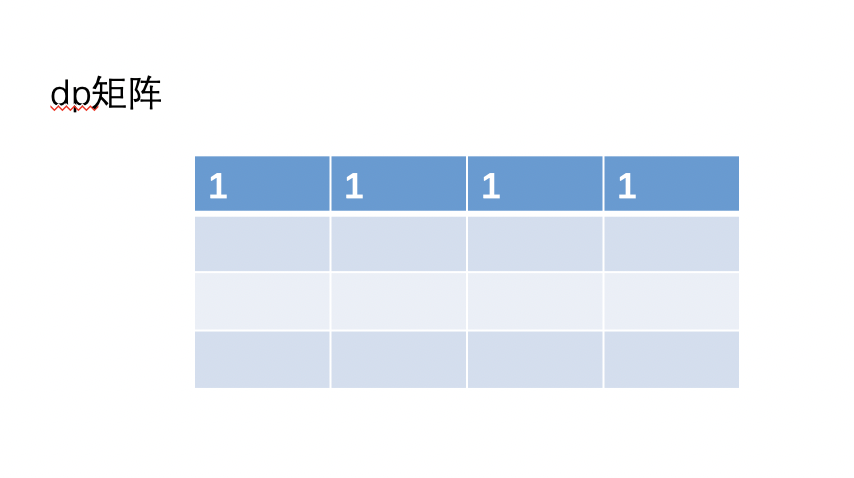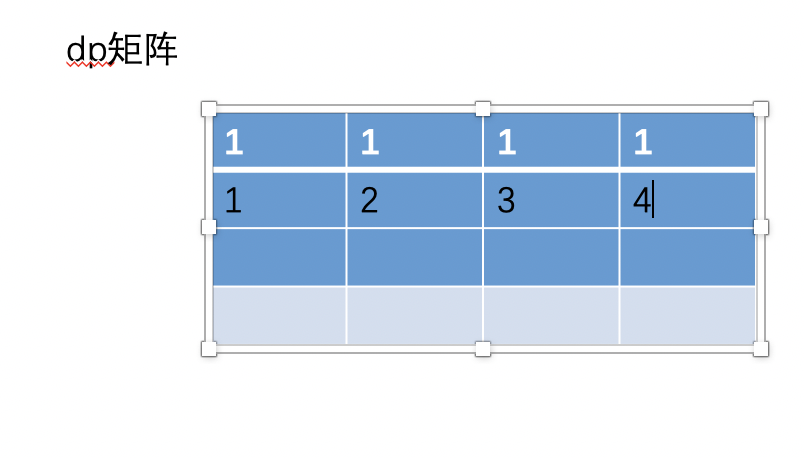1、刚开始初始化第一行，此时 dp[0..n-1] 的值就是第一行的值。2、接着我们来一边填充第二行的值一边更新 dp[i] 的值，一边把第一行的值抛弃掉。

（1）、显然，矩阵(1, 0) 的值相当于以往的初始化值，为 1。然后这个时候矩阵 (0，0）的值不在需要保存了，因为再也用不到了。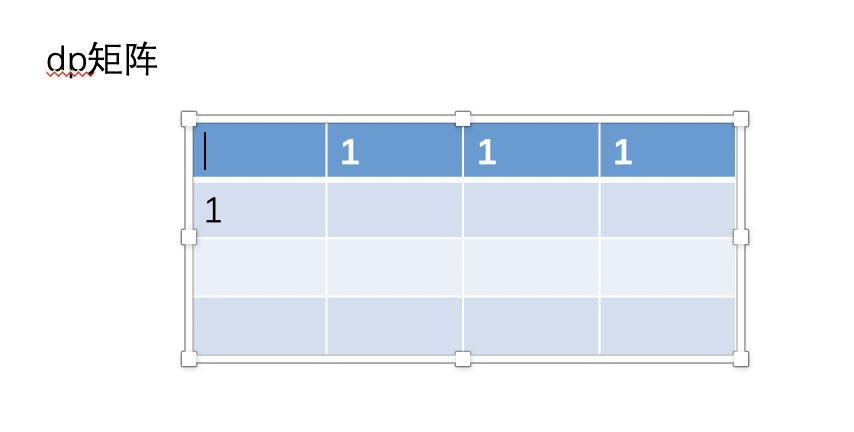（2）、接着继续更新 (1, 1) 的值，根据之前的公式 （i, j) = (i-1, j) + (i, j- 1)。即 （1，1）=（0，1）+（1，0）=2。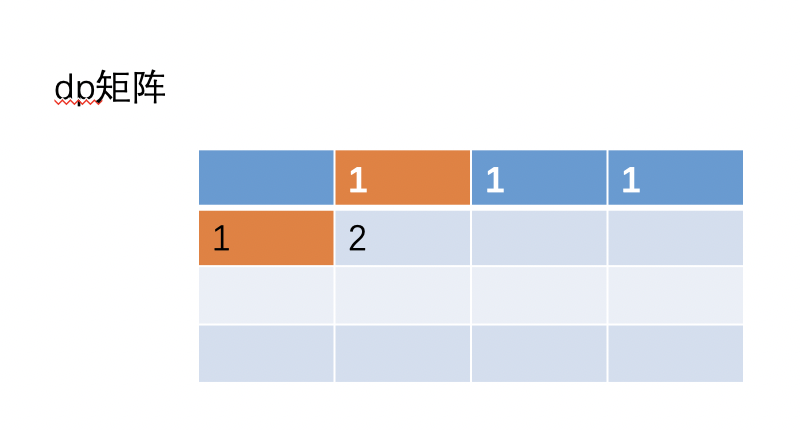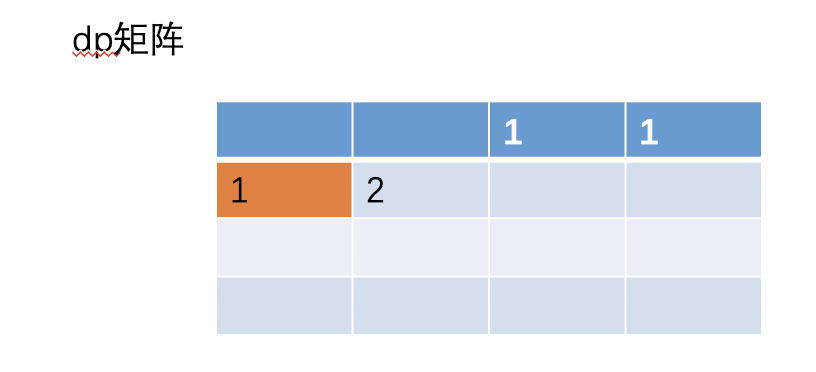(3)、同样的道理，按照这样的模式一直来计算第二行的值，顺便把第一行的值抛弃掉，结果如下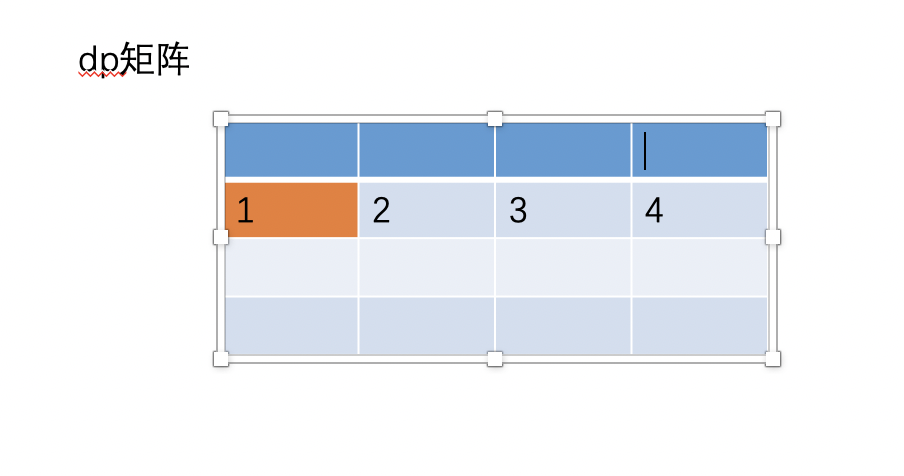dp[i] = dp[i-1] + dp[i]

dp[i-1] 相当于之前的 dp[i-1][j]，dp[i] 相当于之前的 dp[i][j-1]。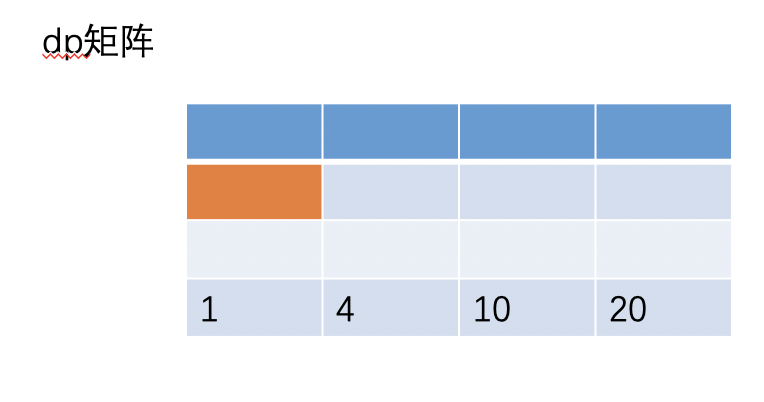public static int uniquePaths(int m, int n) {
if (m <= 0 || n <= 0) {
return 0;
}

int[] dp = new int[n]; //
// 初始化
for(int i = 0; i < n; i++){
dp[i] = 1;
}

// 公式：dp[i] = dp[i-1] + dp[i]
for (int i = 1; i < m; i++) {
// 第 i 行第 0 列的初始值
dp = 1;
for (int j = 1; j < n; j++) {
dp[j] = dp[j-1] + dp[j];
}
}
return dp[n-1];
}


### 案例2：编辑距离

示例：

horse -> rorse (将 'h' 替换为 'r')
rorse -> rose (删除 'r')
rose -> ros (删除 'e')


public int minDistance(String word1, String word2) {
int n1 = word1.length();
int n2 = word2.length();
int[][] dp = new int[n1 + 1][n2 + 1];
// dp[0...n2]的初始值
for (int j = 1; j <= n2; j++)
dp[j] = dp[j - 1] + 1;
// dp[0...n1] 的初始值
for (int i = 1; i <= n1; i++) dp[i] = dp[i - 1] + 1;
// 通过公式推出 dp[n1][n2]
for (int i = 1; i <= n1; i++) {
for (int j = 1; j <= n2; j++) {
// 如果 word1[i] 与 word2[j] 相等。第 i 个字符对应下标是 i-1
if (word1.charAt(i - 1) == word2.charAt(j - 1)){
p[i][j] = dp[i - 1][j - 1];
}else {
dp[i][j] = Math.min(Math.min(dp[i - 1][j - 1], dp[i][j - 1]), dp[i - 1][j]) + 1;
}
}
}
return dp[n1][n2];
}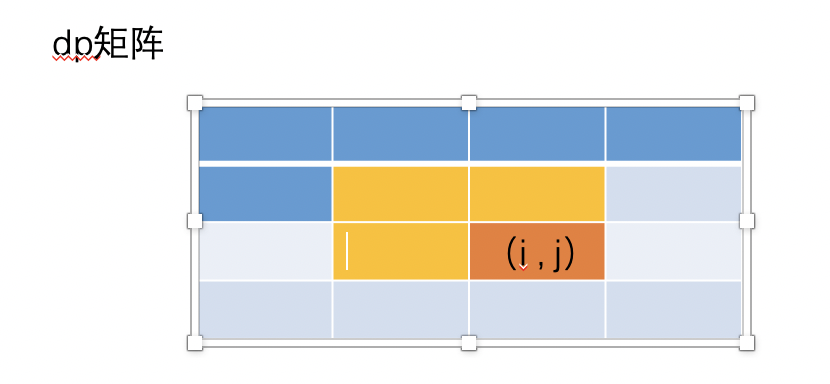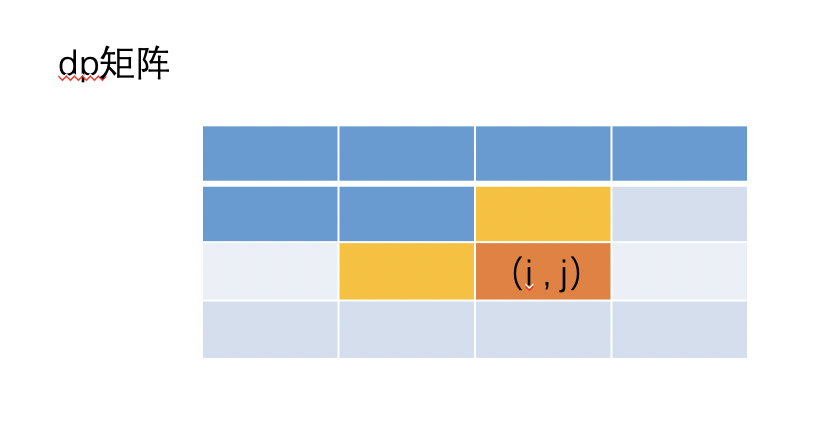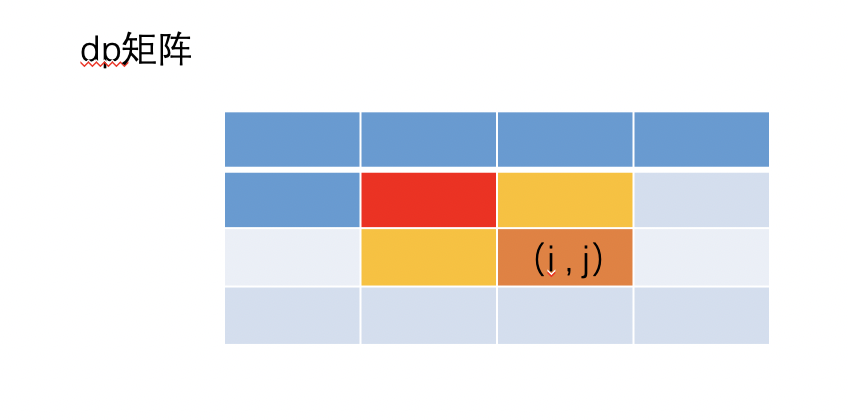dp[i][j] = min(dp[i-1][j] , dp[i-1][j-1] , dp[i][j-1]) + 1


dp[i] = min(dp[i-1], pre, dp[i]) + 1。


##### 代码如下
public int minDistance(String word1, String word2) {
int n1 = word1.length();
int n2 = word2.length();
int[] dp = new int[n2 + 1];
// dp[0...n2]的初始值
for (int j = 0; j <= n2; j++)
dp[j] = j;
// dp[j] = min(dp[j-1], pre, dp[j]) + 1
for (int i = 1; i <= n1; i++) {
int temp = dp;
// 相当于初始化
dp = i;
for (int j = 1; j <= n2; j++) {
// pre 相当于之前的 dp[i-1][j-1]
int pre = temp;
temp = dp[j];
// 如果 word1[i] 与 word2[j] 相等。第 i 个字符对应下标是 i-1
if (word1.charAt(i - 1) == word2.charAt(j - 1)){
dp[j] = pre;
}else {
dp[j] = Math.min(Math.min(dp[j - 1], pre), dp[j]) + 1;
}
// 保存要被抛弃的值
}
}
return dp[n2];
}


### 有收获？希望老铁们来个三连击，给更多的人看到这篇文章

1、点赞，可以让更多的人看到这篇文章
2、关注我的原创微信公众号『苦逼的码农』，第一时间阅读我的文章，已写了 150+ 的原创文章。### 另外推广一波极客时间的福利，顺便给你们暖心的博主助力（极客时间的挺多专栏都写的挺不错哦）。posted @ 2019-11-14 09:03  帅地  阅读(6715)  评论(14编辑  收藏  举报